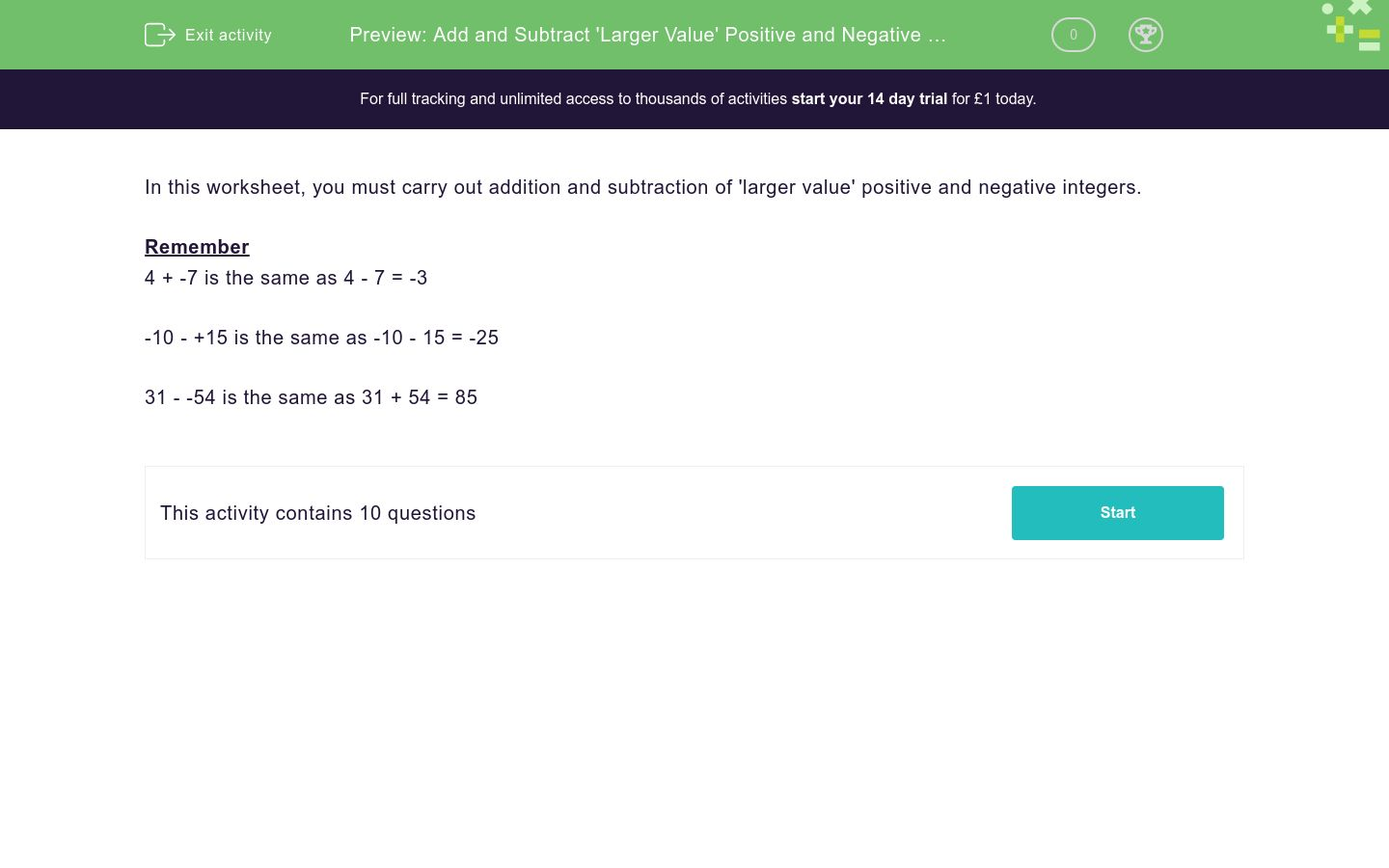# Add and Subtract 'Larger Value' Positive and Negative Integers

In this worksheet, students carry out addition and subtraction of 'larger value' positive and negative integers.Key stage:  KS 3

Curriculum topic:   Number

Curriculum subtopic:   Use Four Operations for All Numbers

Difficulty level:### QUESTION 1 of 10

In this worksheet, you must carry out addition and subtraction of 'larger value' positive and negative integers.

Remember

4 + -7 is the same as 4 - 7 = -3

-10 - +15 is the same as -10 - 15 = -25

31 - -54 is the same as 31 + 54 = 85

Work out:

-87 + -35 = ________

Work out:

-89 + -12 = ________

Work out:

-15 - -9 = ________

Work out:

22 + -61 = ________

Work out:

-21 + -46 = ________

Work out:

85 + -30 = ________

Work out:

-129 - -129 = ________

Work out:

-92 - -79 = ________

Work out:

70 - -55 = ________

Work out:

-19 - -33 = ________

• Question 1

Work out:

-87 + -35 = ________

-122
• Question 2

Work out:

-89 + -12 = ________

-101
• Question 3

Work out:

-15 - -9 = ________

-6
• Question 4

Work out:

22 + -61 = ________

-39
• Question 5

Work out:

-21 + -46 = ________

-67
• Question 6

Work out:

85 + -30 = ________

55
• Question 7

Work out:

-129 - -129 = ________

0
• Question 8

Work out:

-92 - -79 = ________

-13
• Question 9

Work out:

70 - -55 = ________

125
• Question 10

Work out:

-19 - -33 = ________

14
---- OR ----

Sign up for a £1 trial so you can track and measure your child's progress on this activity.

### What is EdPlace?

We're your National Curriculum aligned online education content provider helping each child succeed in English, maths and science from year 1 to GCSE. With an EdPlace account you’ll be able to track and measure progress, helping each child achieve their best. We build confidence and attainment by personalising each child’s learning at a level that suits them.

Get started